# Forchheimer, Non-boussinseq Natural Convection in Porous Media Filled Enclosure

Forchheimer, Non-boussinseq Natural Convection in Porous Media Filled Enclosure

A.M. OsmanH.M. Duwairi

Mechanical Engineering Department, Faculty of Engineering & Technology, 11941 Amman, Jordan

Corresponding Author Email:
Duwairi@ju.edu.jo
Page:
191-196
|
DOI:
http://dx.doi.org/10.18280/ijht.330425
|
Accepted:
|
Published:
31 December 2015
| Citation

OPEN ACCESS

Abstract:

In this work the Forchheimer, non-Boussinesq natural convection heat transfer of water around 4 oC. is analyzed, the following dimensionless parameters are found to describe the problem which are namely the modified Rayleigh number (Raw), the inclination angle (Ф), the aspect ratio of the enclosure (A), and the exponent for non-Boussinesq-approximation (n). It was found that the increasing of the modified Rayleigh number Raw increased the mass flow rates and the buoyancy forces and consequently increases the mean Nusselt number, it was found also that the increasing of the value of the exponent for non-Boussinesq-approximation (n); which physically means a non-linear temperature – density relationship, caused the mean Nusselt number and the dimensionless stream function to decrease to reach their minimum values at n=2, this is due to smaller temperature difference and consequently smaller buoyancy forces. The inclination angle (Ф), has a certain effect on heat transfer and fluid flow as the maximum heat transfer rate is obtained at inclination angels around Ф=30◦-60◦.

(Presented at the AIGE Conference 2015)

Keywords:

Non-Boussinseq, Natural convection, Temperature of 4 oC.

1. Introduction

The flow of homogenous fluids and heat transfer through porous media has been of considerable interest because of numerous applications of flow through porous media. Fluid density usually changes as a function of the fluid’s temperature in a reasonably linear manner. A notable exception is liquid water. Pure water at a pressure of one atmosphere has a maximum density of 999.9720 kg/m3 at 4°C. Above this temperature the density of water decreases as the temperature is increased in a manner similar to other fluids. For temperatures below 4°C, the trend is reversed as density increases with increased temperature, giving rise to a maximum density at the 4°C point.

In previous paper, Duwairi et al.  investigated the problem of transient laminar magneto hydrodynamic free convection over a vertical isothermal plate in water in the temperature range between 20°C and 0°C assuming a linear relationship between fluid density and temperature. It was assumed then that the equation Eq. (1). Can be employed to give the density of pure or saline water instead of the linear-density model used in the Boussinesq-approximation:

$\rho=\rho_{m}\left(1-\beta\left(T-T_{m}\right)^{n}\right)$     (1)

Where $\rho_{m}$ and $\mathrm{T}_{\mathrm{m}}$ are the maximum density and temperature at the given pressure and salinity, β is the coefficient of thermal expansion and n is the exponent. The present paper extends this work by investigating the natural convection of water at 4 °C in a porous media filled rectangular enclosure. To present the result for the Non-Boussinesq approximation along with other parameters which are included in the final system of partial differential equations, the governing continuity, momentum and energy equations are going to non-dimensionlized using suitable stream function and dimensionless temperature ratios and finally solved using finite difference method. The stream lines and isotherms are plotted to obtain flow patterns and temperature fields at different effects of the above parameters as well as the mass and heat fluxes inside the porous enclosure. The boundary conditions selected on the enclosure are two adiabatic and two isothermal walls.

In the literature many studies exists for the mixed convection boundary layer flow about vertical, inclined, and wedge surfaces immersed in a viscous fluid. Kumaran and Pop  who studied the steady free convection from a vertical wall/plate embedded in a porous medium filled with water at 4 °C, Oztop et al.   analyzed the natural convection in right-angle triangular enclosure filled with saturated cold water which has a density maximum. Duwairi and Al-Kablawi  Aldoss et al.  who considered free convection flow past a vertical surface under different boundary conditions. Dasilva et al.  studied the convection heat transfer inside a vertical channel in the presence of heat sources.

Others dealt with free/ mixed convection boundary layer flow of water at 4 ◦c past different geometries with constant or variable surface temperature in a porous medium among those was Guedda et al.   who investigated the two-dimensional mixed convection boundary-layer flow over a vertical flat plate embedded in a porous medium saturated with a water at 4 °C, Khan and Gorla  studied the mixed convection of water at 4 ◦c along a wedge in a porous media, also Ling et al.  considered the steady mixed convection boundary-layer flow over a vertical impermeable surface in a porous medium saturated with water close to its maximum density. In all these studies the free/mixed convection of water at 4 ◦c for external flow were investigated either for flow in a plain or a porous media, but little attention is given to the internal flow heat transfer problem due to complexity in solving for the pressure gradients inside different conduits.

2. Mathmatical Formulation and Analysis

The geometry considered is an inclined rectangular enclosure, which embedded in a fluid-saturated porous media as shown in fig. (1). One wall of the enclosure is kept at uniform high temperature and the opposite is kept at a uniform low temperature. The other two walls of the enclosure are adiabatic, i.e., it is assumed that no heat is transferred into or out of walls. The governing equations can be written as Duwairi and Damseh :

## 1.png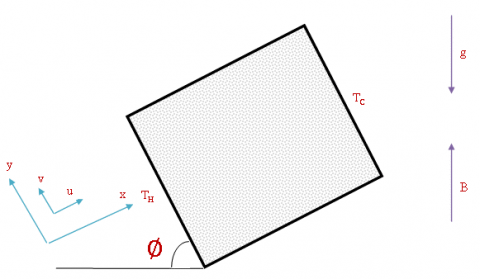Figure 1. Schematic of physical model and coordinate system

$\frac{\partial u}{\partial x}+\frac{\partial v}{\partial y}=0$     (1)

The momentum equation in x and y-directions can be written as:

$\frac{\mu}{K}\left(\frac{\partial u}{\partial y}-\frac{\partial v}{\partial x}\right)+\frac{C_{f} \rho}{\sqrt{K}} \frac{\partial u^{2}}{\partial y} =n \rho \beta g\left(T-T_{C}\right)^{n-1}\left(\frac{\partial T}{\partial y} \cos \Phi-\frac{\partial T}{\partial x} \sin \Phi\right)$    (2)

The energy equation:

$u \frac{\partial T}{\partial x}+v \frac{\partial T}{\partial y}=\frac{k}{\rho c_{p}}\left(\frac{\partial^{2} T}{\partial x^{2}}+\frac{\partial^{2} T}{\partial y^{2}}\right)$   (3)

Where x and y are the horizontal and vertical coordinates, and the corresponding velocities are u and v respectively, the gravitational acceleration g is acting downward in the direction opposite to the y coordinate. The Darcy effect is introduced through the term $\left(\frac{\mu u}{K}\right)$  in the momentum equation in x and y-directions. The non-Darcy effect is introduced through the Forchheimer term $\left(\frac{\rho C_{F}}{\sqrt{K}} u^{2}\right)$ in the momentum equation in x and y-directions. The non-Boussinseq term effects on convection heat transfer is introduced into the governing equations through two terms $\left(\rho g \beta\left(T-T_{c}\right) \cos \varphi\right)$  and $\left(\rho g \beta\left(T-T_{c}\right) \sin \varphi\right)$  in the momentum equation raised to the power n. For n=1 the problem is the traditional Boussinesq convection heat transfer problem, for n>1 the problem is the non-Boussinesq convection heat transfer problem. The boundary conditions on the solution for flow in the enclosure are:

$\begin{array}{ll}T=T_{H} & \text { at } x=0 \\ T=T_{c} & \text { at } x=W \\ \frac{\partial T}{\partial y}=0 & \text { at } y=0 \text { and } y=H \\ u=0 & \text { at } x=0 \text { and } x=W \\ v=0 & \text { at } y=0 \text { and } y=H\end{array}$   (4)

The solution will be obtained in terms of the stream function. The stream function is defined by:

$u=\frac{\partial \psi}{\partial y}, \quad v=-\frac{\partial \psi}{\partial x}$  (5)

Before discussing the solution to the above pair of equations, they will be written in dimensionless form. For this purpose, the following dimensionless variables are defined:

$X=\frac{x}{W}, \quad Y=\frac{y}{W}, \quad \Psi=\frac{\psi}{\alpha_{a}}, \quad \theta=\frac{T-T_{c}}{T_{H}-T_{c}}$   (6)

In terms of dimensionless stream function, the continuity, momentum, energy equations:

$\frac{\partial^{2} \Psi}{\partial X \partial Y}-\frac{\partial^{2} \Psi}{\partial X \partial Y}=0$    (7)

$\frac{\partial^{2} \Psi}{\partial X^{2}}+\frac{\partial^{2} \Psi}{\partial Y^{2}}+2 F r \frac{\partial \Psi}{\partial Y} \frac{\partial^{2} \Psi}{\partial Y^{2}}=R a_{w} \theta^{n-1}\left(\frac{\partial \theta}{\partial Y} \cos \varphi-\frac{\partial \theta}{\partial X} \sin \varphi\right)$   (8)

$\frac{\partial \Psi}{\partial Y} \frac{\partial \theta}{\partial X}-\frac{\partial \Psi}{\partial X} \frac{\partial \theta}{\partial Y}=\frac{\partial^{2} \theta}{\partial X^{2}}+\frac{\partial^{2} \theta}{\partial Y^{2}}$    (9)

Here $R a_{W}=\frac{n \operatorname{Kg} \beta\left(T_{H}-T_{c}\right)^{n}}{\alpha_{a} v}, \quad F r=\frac{C_{F} \alpha_{a}}{v W}$    (10)

Where $\mathrm{Ra}_{\mathrm{w}}$  is the Darcy-modified Rayleigh number based on the enclosure width W. Fr is the Forchheimer number based on the enclosure width W. In terms of dimensionless variables defined in Eq. (6), the boundary conditions are:

$\begin{array}{ll}\theta=1 & \text { at } X=0 \\ \theta=0, & \text { at } X=1 \\ \frac{\partial \theta}{\partial Y}=0 & \text { at } Y=0 \text { and } Y=A\end{array}$    (11)

Here $A=H / W$  is the so-called aspect ratio of the enclosure.

All wall surfaces Ψ=0    (12)

A finite difference numerical procedure is used to solve the governing equations (7-10) with the corresponding boundary conditions (11 & 12), an iterative procedure is used in which all the variables at the nodal points are first suggested, updated values are than obtained by applying the governing equations and the process is repeated until convergence is attained. To check accuracy of the predicted results, a comparison is done with those of Oosthuizen and Naylor  for the case of absent magnetic field strength and the both results are shown in table.1. It is clear from these results that they are in complete agreement.

3. Results and Discussion

A numerical study was performed to examine the steady-state, laminar convection heat transfer problem inside a porous media filled inclined rectangular enclosures with the parameters as power exponent of non-Boussinseq equation, modified Darcy Rayleigh number, inclination angle and the aspect ratio of the enclosure. The left-hand side wall is hot and the right-hand side wall is cold while the other two walls of the enclosure are adiabatic. The Finite difference scheme is used to solve the momentum and energy equations. The dimensionless streamlines and isotherms are plotted to obtain flow patterns and temperature fields at different effects of all parameters above. Also, the Nusselt numbers and the center dimensionless streams are plotted.

Table 1. Comparison between predicted results and those obtained by Oosthuizen and Naylor 

 $\varphi$ Oosthuizen and Naylor  $N u$ Presented Results $N u$ 00 1 1 300 2.75 2.7450 900 2.5 2.5084 1800 1 1

The effect of the modified Rayleigh number on the dimensionless streamlines and isotherms patterns was studied for different values of the exponent for non-Boussinesq-approximation, (n=1and n=2).

Figure (2) illustrates the effect of the modified Rayleigh number in the momentum equation on the dimensionless streamlines and isotherms patterns for various values of Raw, with other parameters unchanged at Ф=30◦, A=1, Fr=0.01 and (n=1and n=2). Comparing results obtained for n=1 with those obtained for n=2, gives a better understanding to the combined effect of both parameters (“Raw” and “n”).

For small values of Rayleigh number i.e. at Raw=1 Fig. (2-a) the hot fluid along the left wall and the cold fluid along the right hand side wall has relatively small value dimensionless stream function of fluid flow, which is 0.04 at the center of the enclosure and the heat transfer becomes by conduction, it was obtained to be 0.06 for Raw=1and n=1. As the Raw number is increased through Raw=50 to reach the maximum value at Raw=100 Fig.(5.4- b&c) the value of dimensionless stream function of fluid flow increases to reach up to 6 at the center of the enclosure. From the results obtained for n=1 we can see that the maximum value at Raw=100 is about 10.And in both cases it can be seen that the gradual increase of the Raw from Raw=1 to Raw=100 has a recognized effect of the flow temperature (dimensionless temperature). The increase in the exponent “n” while keeping the value of Raw constant mans small rates of buoyancy forces, so in actual increasing the exponent “n” will result in an increase in the dimensionless temperature flow rates, and defines higher temperature inside the enclosure, this is due to the high buoyancy force effect on the fluid circulation. Also the relation between the variation of mean Nusselt number and the dimensionless stream function at the center of the enclosure and the inclination angel for an enclosure with various values of Rayleigh number for a square enclosure i.e. A=1 and Fr=0.01 for the exponent for non-Boussinesq-approximation n = 1, is illustrated by Figure (5).

## 2a.png## 2a1.png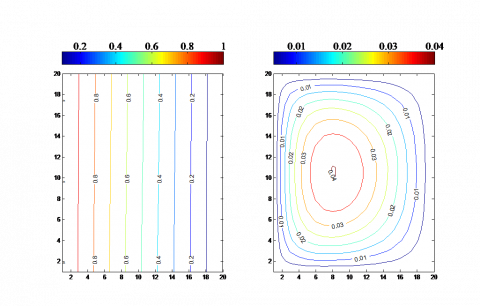(a)

## 2b1.png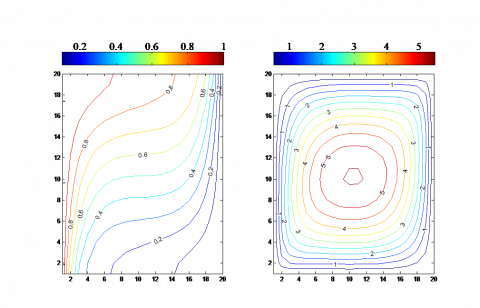## 2b2.png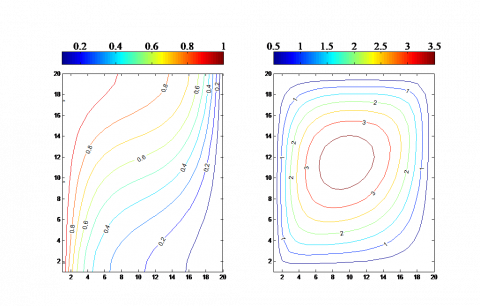(b)

## 2c1.png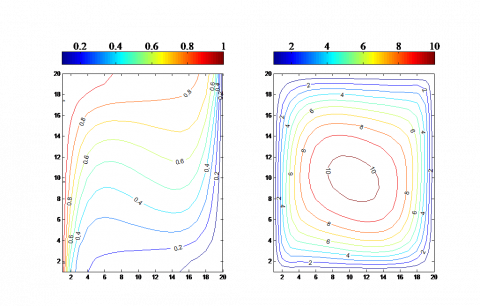## 2c2.png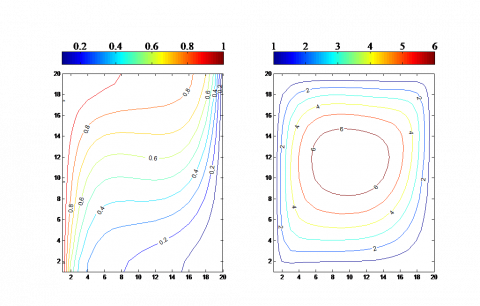(c)

Figure 2. Typical dimensionless streamline and dimensionless temperature patterns for various Darcy-modified Rayleigh number, (a) Raw=1, (b) Raw=50, (c) Raw=100, for Ф=30°, A=1, Fr=0.01 and n=1

## 3.png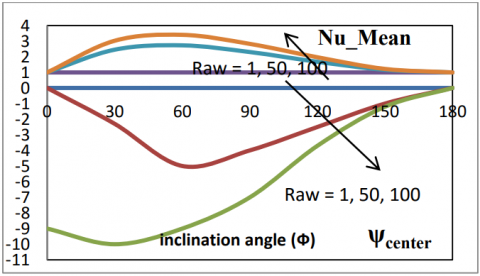Figure 3. Variation of mean Nusselt number and the dimensionless center-stream function with the inclination angel for an enclosure with various values of Raw at A=1 Fr=0.01 and n=1

## 4a1.png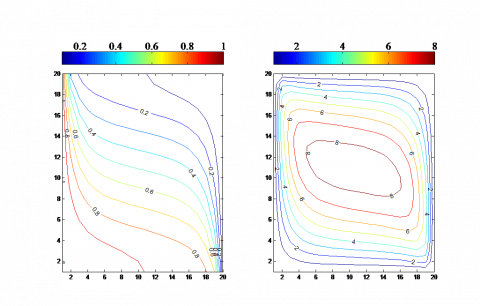## 4a2.png(a)

## 4b1.png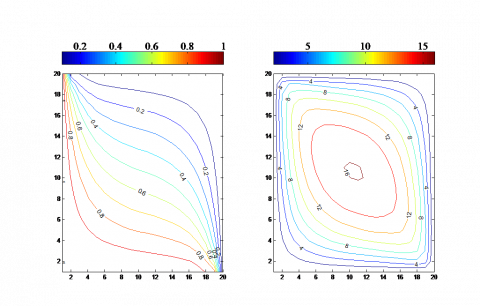## 4b2.png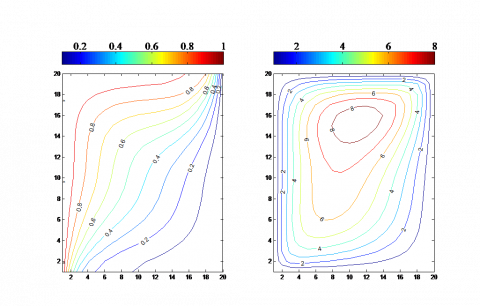(b)

## 4c1.png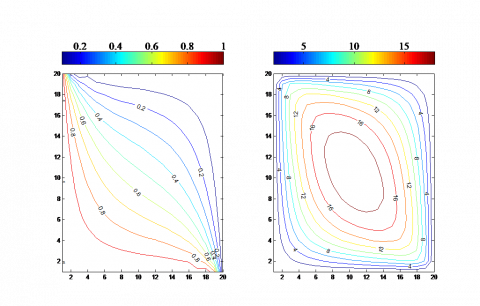## 4c2.png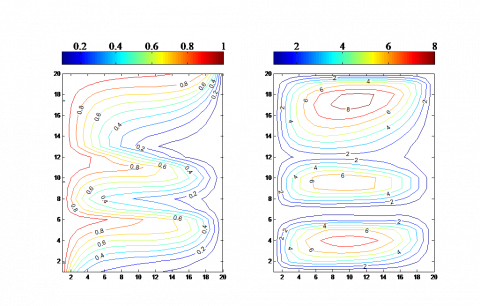(c)

Figure 4. Typical dimensionless streamline and dimensionless temperature patterns for various aspect ratio of the enclosure, (a) A=1, (b) A=3 (c) A=5, for Ф=30°, Raw=100, Fr=0.01 and n=2

## 5.png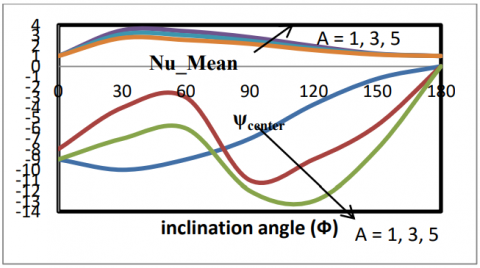Figure 5. Variation of mean Nusselt number and the dimensionless center-stream function with the inclination angel for an enclosure with various values of the enclosure’s aspect ratio (A) at n=1 Fr=0.01 and Raw=100

## 6a1.png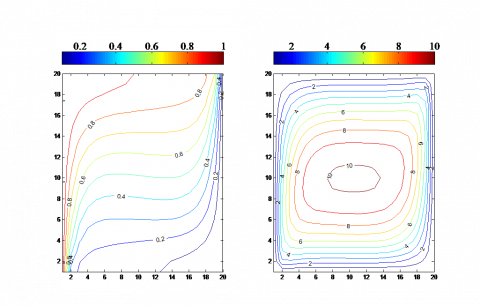## 6a2.png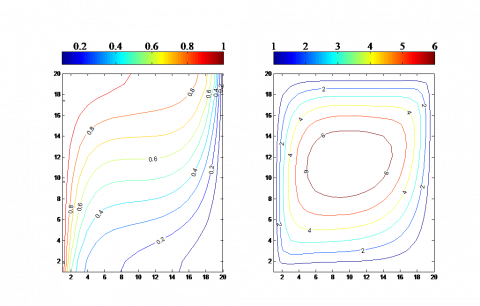(a)

## 6b1.png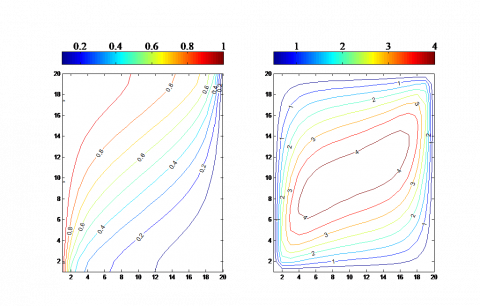## 6b2.png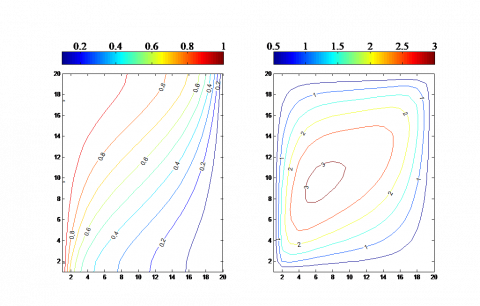(b)

## 6c1.png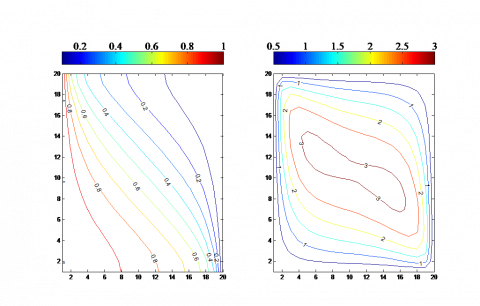## 6c2.png(c)

Figure 6. Typical dimensionless streamline and dimensionless temperature patterns for various inclination angel of the enclosure, (a) Ф=45°, (b) Ф=90°, (c) Ф=180°, for Raw=100, A=1, Fr=0.01 and n=2

## 7.png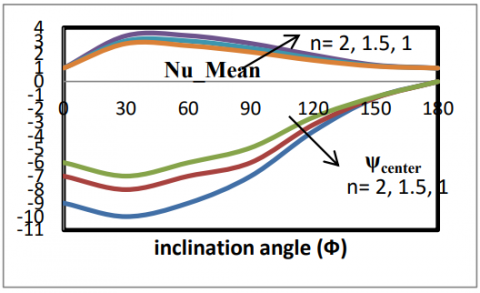Figure 7. Variation of mean Nusselt number and the dimensionless center-stream function with the inclination angel for an enclosure with various values of (n) at A=1 Fr=0.01 and Raw=100

Nomenclature
 u The velocity in x direction. [m/ s] v The velocity in y direction. [m/ s] $\mathrm{T}_{\mathrm{c}}$ The cold wall temperature. [K] $\mathrm{T}_{\mathrm{H}}$ The hot wall temperature. [K] g Gravitational acceleration. [m/ s2] K Permeability. [m2] $C_{F}$ Forchheimer constant. n Power Eexponent in non-Boussinseq equation P Pressure [Pa] T Temperature. [K] W The length in x-direction. [m] H The length in y-direction. [m] ka Thermal conductivity of the porous media and fluid.   [W/m.K] $\mathrm{C}_{\mathrm{p}}$ Specific heat at constant pressure. [kJ/kg.K] Fr Forchheimer’s number. $\mathrm{Ra}_{\mathrm{w}}$ The Darcy- Rayleigh number. A The aspect ratio of the enclosure. Greek symbols $\phi$ The inclination angle of the enclosure. [degrees] $\mu$ Absolute viscosity. [kg/m.s] $\rho$ The fluid density. [kg/ m3] $\rho_{o}$ The fluid density at some reference temperature, [kg/ m3 ] $\beta$ Coefficient of thermal expansion. [ $\mathrm{K}^{-1}$ ] $\psi$ Streamline function. $\Psi$ Dimensionless stream function. $\alpha_{\mathrm{a}}$ Thermal diffusivity. [m2/ s]
References

1. Duwairi, H.M., R.A. Damseh and B. Tashtoush, “Transient non-boussinseq magnetohydrodynamic free convection flows over vertical surfaces,” Int. J. of Fluid Mechanics Research, vol. 33, no. 2, pp. 1485-1493, 2006. DOI: 10.1615/InterJFluidMechRes.v33.i2.20.

2. Kumaran V., and Pop. I, “Steady free convection boundary flow over a vertical flat plate embedded in porous medium filled with water at 4 oC,” Int. J. of Heat and Mass Transfer, vol. 49, no. 17, pp. 3240-3252, 2006. DOI: 10.1016/j.ijheatmasstransfer.2006.01.026.

3. Oztop, H.F., Farol, Y. and Pop, I., “Investigation of Natural Convection in Traingular enclosure filled with Porous Media Saturated with Water near 4 oC,” Energy Conversion and Management, vol. 50, no. 6, pp. 1473-1480, 2009. DOI: 10.1016/j.enconman.2009.02.023.

4. Duwairi, H.M. and Kablawi, Y., “MHD-conjugate mixed convection heat transfer over a vertical hollow cylinder embedded in a porous medium,” Int. J. Heat and Technology, vol. 24, no. 1, pp. 123-128, 2006.

5. Aldoss, T.K., Jarrah, M.A. and Duwairi, H.M., “Wall effect on mixed convection from horizontal surfaces with a variable surface heat flux,” The Canadian Journal of Chemical Engineering, vol. 72, no. 1,pp. 35-42, 1994. DOI: 10.1002/cjce.5450720106.

6. Alexandre K. da Silva, Giulio Lorenzini, Adrian Bejan, “Distribution of heat sources in vertical open channels with natural convection,” International Journal of Heat and Mass Transfer, vol. 48, no. 8, pp. 1462 – 1469, 2005.

7. Guedda, M., Aly, E. H. and Ouahsine, A., “Analytical and ChPDM analysis of MHD mixed convection over a vertical flat plate embedded in a porous medium filled with water at 4 oC,” Applied Mathematical Modeling, vol. 35, no. 10, pp. pp. 5182-5197, 2011. DOI: 10.1016/j.apm.2011.04.014.

8. Khan, W.A. and Gorla, R.S., “Mixed convection of water at 4 oC along a wedge with variable surface flux in

a porous medium,” Transport in Porous Media, vol. 83, no. 2, pp. 413-424, 2009.

9. Ling, S.C., Nazar, R., Pop, I., and Merkin, J.H., “Mixed convection boundary layer flow in a porous medium filled with water close to its maximum density,” Transport in Porous Media, vol. 76, no. 1, pp. 139-151, 2008.

10. Duwairi, H.M. and Damseh R.A., “Thermophoresis particle deposition-thermal radiation interaction on mixed convection from vertical surfaces embedded in porous medium,” Canadian Journal of Physics, vol. 87, no. 2, pp. 161-167, 2009. DOI: 10.1139/P08-128.

11. Oosthuizen, P.H. and and Naylor, D., An Introduction to Convective Heat Transfer Analysis, McGraw-Hill, 1991.